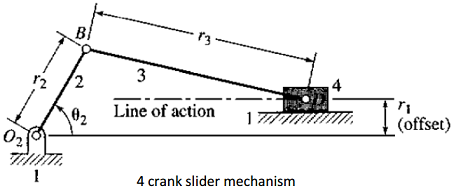### Find the displacement, velocity and acceleration

Assignment Help Mechanical Engineering
##### Reference no: EM13863211

Q.1 Figure shows a four bar linkage mechanism. For each configuration shown in table 1, determine the angular displacements, angular velocities and angular accelerations of links 3 and 4 graphically and analytically . Use the Excel sheet discussed in the lecture to help you do the analytical solution.r1 (cm) r2 (cm) r3 (cm) r4 (cm) θ2 (degrees) θ2 (rad/sec) θ2 (rad/sec2) (a) 5.0 1.0 4.0 3.0 30 30 0 (b) 8.0 2.0 5.0 4.0 60 45 0 (c) 10.0 32.0 20.0 20.0 120 -50 40 (d) 5.0 1.0 6.0 4.0 210 100 -40

Q.2
Use figure 2 and the information provided in table 2 to solve this question For each configuration shown in table 2, determine the angular displacement, angular velocity and angular acceleration of link 3. Also find the displacement, velocity and acceleration of link4r1 (cm) r2 (cm) r3 (cm) θ2 (degrees) θ2 (rad/sec) θ2 (rad/sec2) (a) 0 5.0 10.0 30 50 0 (b) 0 3.0 4.0 60 45 0 (c) 2.0 3.0 6.0 120 -50 40 (d) -2.0 4.0 10.0 150 100 -40

### Write a Review